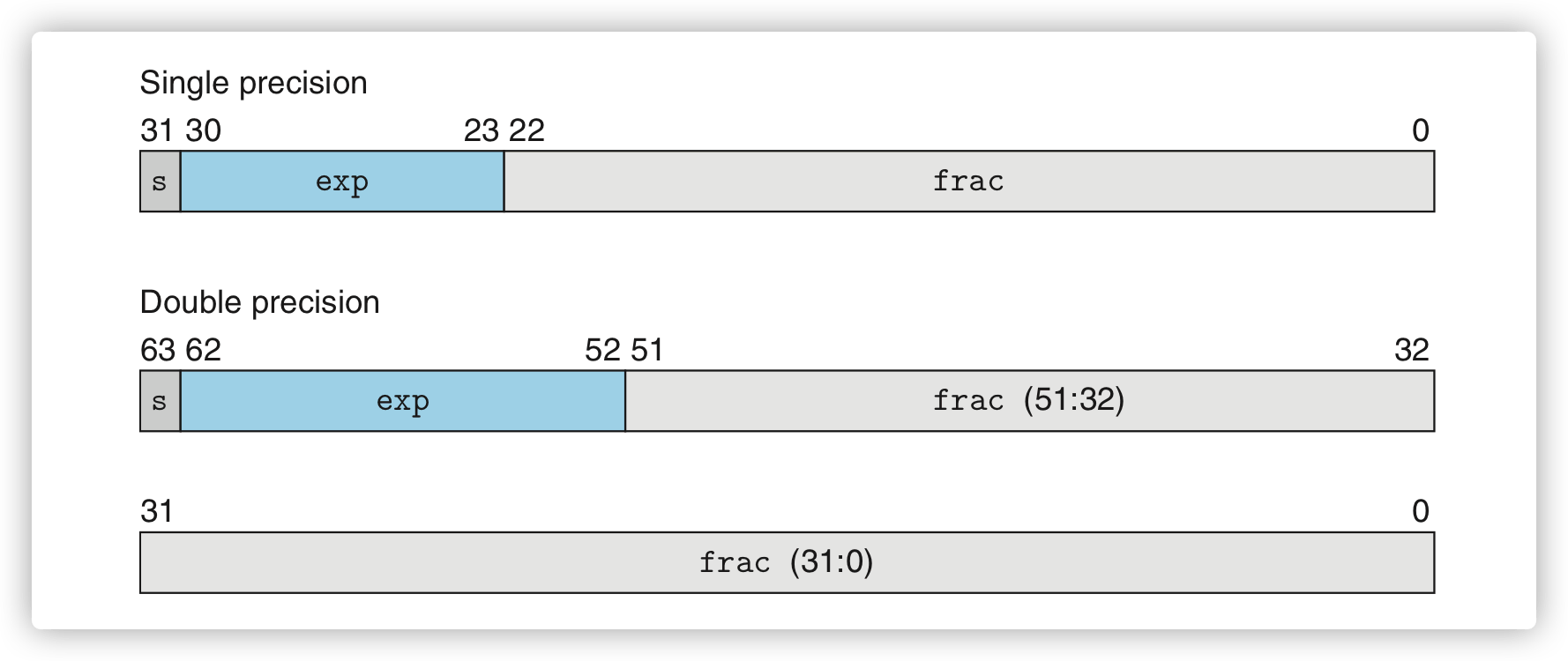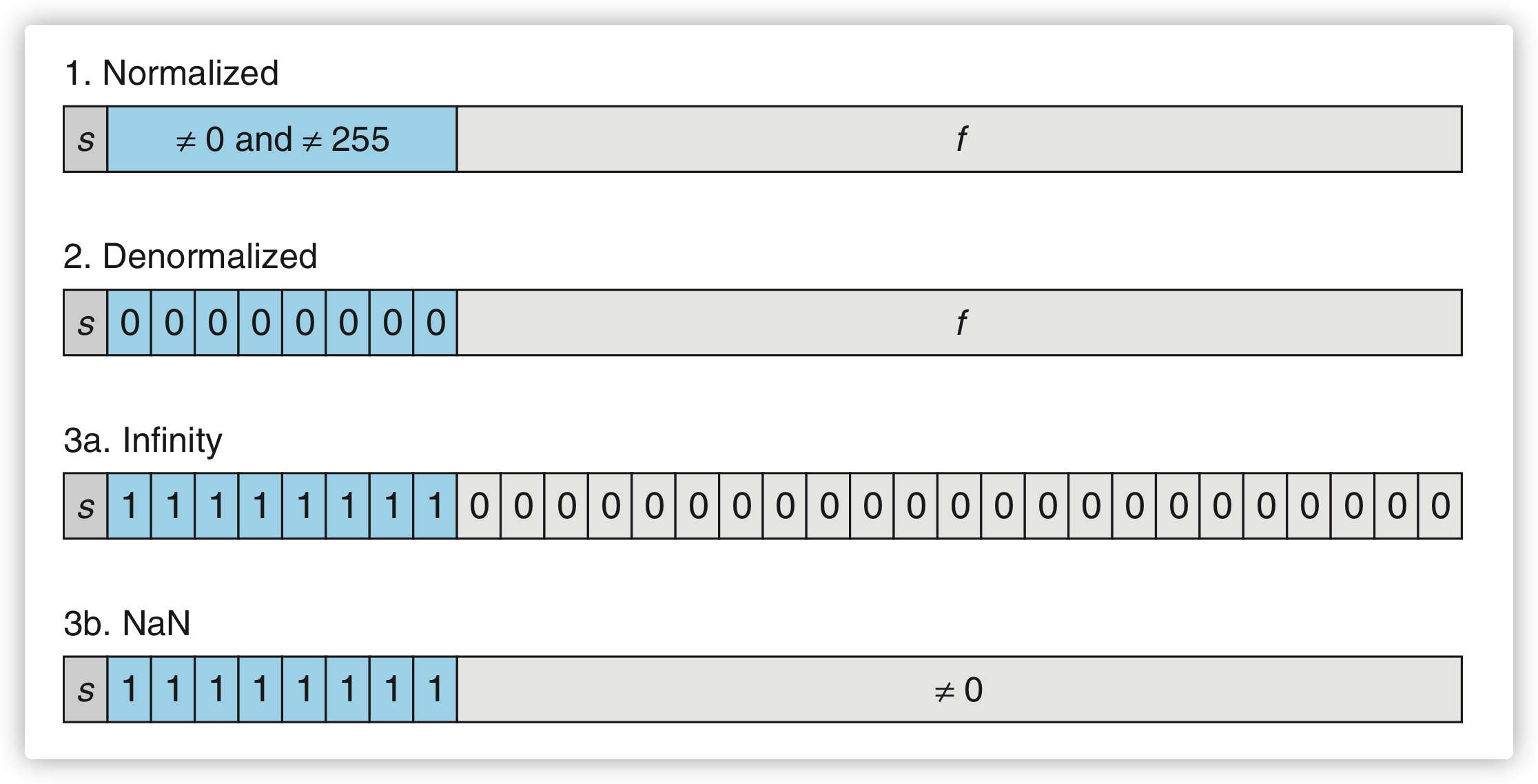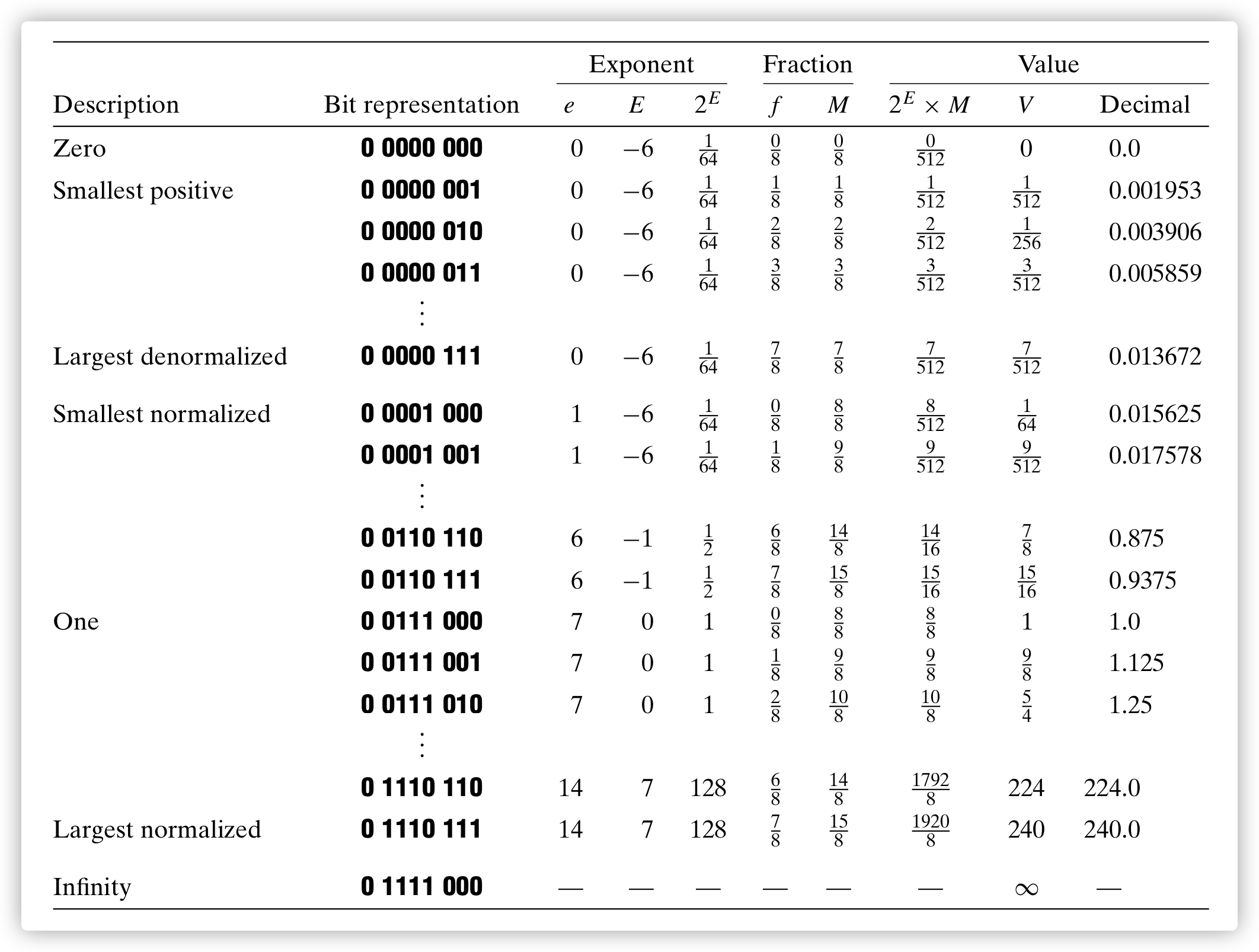# [CSAPP] 计算机如何表示浮点数

 1 2  >>> 0.1 + 0.2 0.30000000000000004 

## 二进制小数

$d = \displaystyle\sum_{{ i = -n}}^m 10^i × d_i$

$b = \displaystyle\sum_{{ i = -n}}^m 2^i × b_i$

## IEEE 754 浮点数

IEEE 754 浮点数标准是如今最流行和通用的浮点数表示标准。它用 $V = (−1)^s × M × 2^E$ 的形式来表示一个数字：

• 符号位 $s$ 决定该数字是正数（$s=0$）还是负数（$s=1$）。数值 0 是一种特殊的情况，我们会在之后解释。
• 有效数 $M$ 是一个二进制小数，它的取值范围为 1 和无限趋近于 2 之间，或者为 0 和无限趋近于 1 之间。具体处于哪个取值范围由指数部分的比特位是否全部为 0 来决定。
• 指数 $E$ 可能为正数也可能为负数，通过 2 的指数幂对最终表示的浮点数进行加权。• 最左边的单个比特位 s 代表符号位 $s$ 的值。
• 由 k 个比特位构成的指数部分 exp $= e_{k-1}...e_1e_0$ 对指数 $E$ 进行编码。
• 由 n 个比特位构成的小数部分 frac $= f_{n-1}...f_1f_0$ 对有效数 $M$ 进行编码。### 非规范化的值（Denormalized）

• 一是为了表示 0，由于规范化值中 $1 <= M < 2$，因此不管指数部分取什么值，所表示的数字 $V$ 都不可能为 0。而在非规范化值中 $0 <= M < 1$，当 M 的值为 0 时，所表示的数字 $V$ 也为 0，此时指数部分和小数部分的所有比特位均为 0。在 IEEE 标准中，取决于此时符号位 $s$ 的值，0 也有正负符号。当 $s=0$ 时，用来表示该浮点数的所有比特位全部为 0， $V = +0.0$；当 $s=1$ 时，$V = -0.0$。在 IEEE 标准中， +0.0 和 -0.0 在某些场景下是不同的。
• 二是为了表示非常趋近于 0 的数。相比规范化值，非规范化表示能够表示更加接近于 0 的数字，且在表示的值非常趋近于 0 时，这些值的分布能保持相对均匀，这种能力也叫「渐进下溢」。

### 示例1. 虽然使用了不同的计算方式，但非规范化值可以平滑地过渡到规范化值，如上最大的非规范化值为 $\frac{7}{512}$，而最小的规范化值为 $\frac{8}{512}$。
2. 随着浮点数的递增，其比特位表示所解释地无符号整数也随之递增，因此我们可以用相对简单的无符号整数的排序规则来对浮点数进行排序，这并非巧合，而是有意设计的。